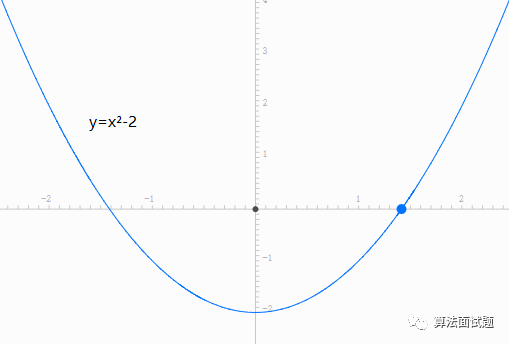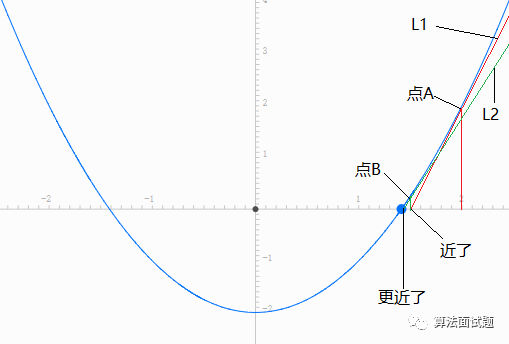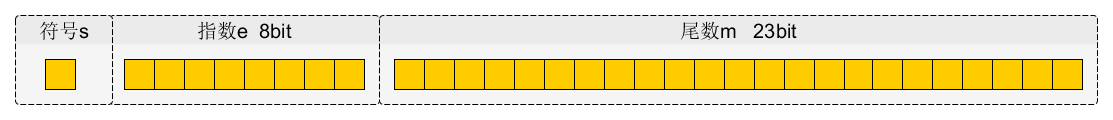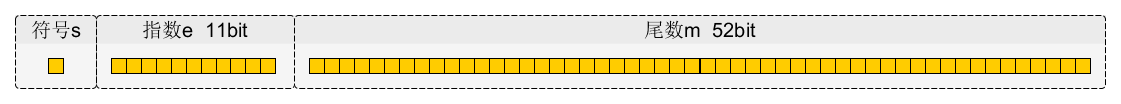# 二分法

[1, 2]
[1, 1.5]
....


# 牛顿迭代法

y = x^2 - 2 的图像## 逼近的过程

### 取 x=1.5# 实现源码

## js - 牛顿迭代法

//求n的算术平方根，参数n不能为负数
function sqrt(n) {
//当n>=1时，从n开始迭代；
//当n<1时，从1开始迭代
let res = n >= 1 ? n : 1;
while(res * res - n > 1e-8)
res = 0.5 * (res + n / res);
return res;
}


## c - 更高效的计算方式

java 的 Math.sqrt() 实际调用的是 c 实现。

• java
/**
* Returns the correctly rounded positive square root of a
* {@code double} value.
* Special cases:
* <ul><li>If the argument is NaN or less than zero, then the result
* is NaN.
* <li>If the argument is positive infinity, then the result is positive
* infinity.
* <li>If the argument is positive zero or negative zero, then the
* result is the same as the argument.</ul>
* Otherwise, the result is the {@code double} value closest to
* the true mathematical square root of the argument value.
*
* @param   a   a value.
* @return  the positive square root of {@code a}.
*/
public static native double sqrt(double a);

• 雷神III 中代码

float Q_rsqrt( float number ) {
long i; float x2, y; const float threehalfs = 1.5F;
x2 = number * 0.5F;
y = number;
i = * ( long * ) &y; // evil floating point bit level hacking
i = 0x5f3759df - ( i >> 1 ); // what the fuck?
y = * ( float * ) &i;
y = y * ( threehalfs - ( x2 * y * y ) ); // 1st iteration
// y = y * ( threehalfs - ( x2 * y * y ) ); // 2nd iteration, this can be removed
#ifndef Q3_VM #
ifdef __linux__ assert( !isnan(y) ); // bk010122 - FPE?
#endif
#endif return y;
}


# 0x5f3759df 是什么？

## 结论

(-1)^S * (1+M/2^23) * 2^(E-127)

# 计算机的浮点数表示

## float

float 类型在内存中占用的位数为: 1+8+23=32bits## double

double 类型在内存中占用的位数为: 1+11+52=64bits## 例子

1.00001001000101010101000。

## 表示

x=(−1)^s × (1.M) × 2^E−127

x=(−1)^s × (1.M) × 2^E−1023

(1) 首先转化为2进制表示

−3.75=−(2+1+1/2+1/4)=−1.111×2^1


(2) 整理符号位并进行规格化表示

−1.111×21=(−1)(1)×(1+0.1110 0000 0000 0000 0000 000)×2^1


(3) 进行阶码的移码处理

(−1)^(1)×(1+0.1110 0000 0000 0000 0000 000)×2^1

=(−1)^(1)×(1+0.1110 0000 0000 0000 0000 000)×2^128−127


# 简化浮点数公式

(-1)^S * (1+M/2^23) * 2^(E-127) 这个公式比较复杂，我们简化一下。

∵ 符号位在 y=x^(-1/2) 的两端都是 0(正数)，可以省去。 ∴ 公式简化为 (1+m) * 2^e

## 案例

7717519 + 120 * 2^23
= 1014350479
= 0X3C75C28F


# 平方根倒数的推导

log2(y) = -1/2 * log2(x)





TODO…

• 牛顿迭代

• 计算机浮点数表示